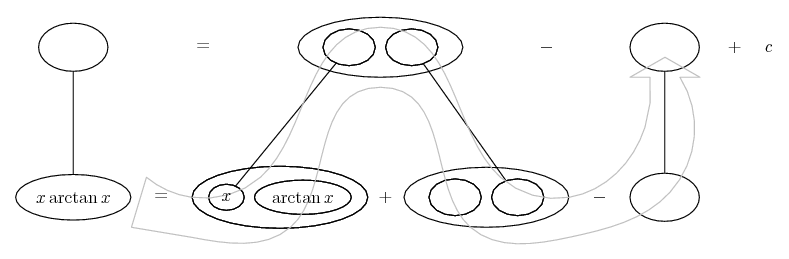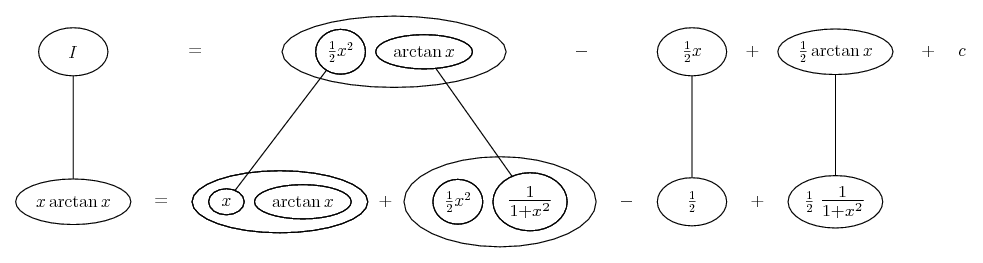# Thread: how do i integrate and go about doing this?

1. ## how do i integrate and go about doing this?

don't know hot to integrate and do this question. I integrated it by parts then got to integrate (x^2/ 2 + 2x^2) again..2. Not sure what you mean, but parts is right (for question a). Integrate x, differentiate arctan x, then integrate 1/2 x^2 all over 1 + x^2, which is equal to

$\displaystyle \frac{1}{2}\ \frac{1 + x^2 - 1}{1 + x^2}$

which you can split into

$\displaystyle \frac{1}{2}\ -\ \frac{1}{2}\ \frac{1}{1 + x^2}$

Just in case a picture helps...More in a tic

3.Originally Posted by tom@ballooncalculusNot sure what you mean, but parts is right (for question a). Integrate x, differentiate arctan x, then integrate 1/2 x^2 all over 1 + x^2, which is equal to

$\displaystyle \frac{1}{2}\ \frac{1 + x^2 - 1}{1 + x^2}$

which you can split into

$\displaystyle \frac{1}{2}\ -\ \frac{1}{2}\ \frac{1}{1 + x^2}$

Just in case a picture helps...More in a tic
how did you go about integrating 1/2 x^2 all over 1 + x^2, ?

4.Originally Posted by mistry88how did you go about integrating 1/2 x^2 all over 1 + x^2, ?
By re-writing the numerator in order to split it as shown above... and now below...Spoiler:... is the product rule. Straight lines differentiate downwards (integrate up) with respect to x.
_________________________________________

Don't integrate - balloontegrate!

Balloon Calculus; standard integrals, derivatives and methods

Balloon Calculus Drawing with LaTeX and Asymptote!

5. re writing the numerator??

sorry about my lack of knowledge6. You know, the top bit.

$\displaystyle \frac{1}{2}\ \frac{x^2}{1 + x^2}$

$\displaystyle =\ \frac{1}{2}\ \frac{1 + x^2 - 1}{1 + x^2}$

$\displaystyle =\ \frac{1}{2}\ \frac{1 + x^2}{1 + x^2}\ -\ \frac{1}{2}\ \frac{1}{1 + x^2}$

$\displaystyle =\ \frac{1}{2}\ -\ \frac{1}{2}\ \frac{1}{1 + x^2}$

It's a trick to by-pass long division, which you should nonetheless know.

#### Search Tags

integrate